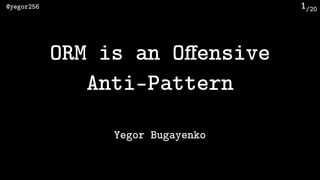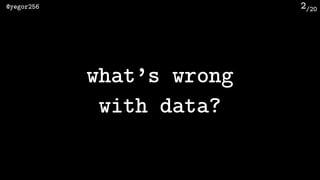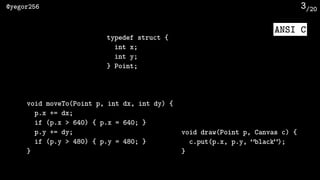Successfully reported this slideshow.

# ORM is an Offensive Anti-Pattern

0

Share×
1 of 24
1 of 24

# ORM is an Offensive Anti-Pattern

0

Share

Øredev 2016; Malmö, Sweden; 9 November 2016; video is here: https://www.youtube.com/watch?v=03PXmPc7Q3g

Øredev 2016; Malmö, Sweden; 9 November 2016; video is here: https://www.youtube.com/watch?v=03PXmPc7Q3g

## More Related Content

### Related Books

Free with a 14 day trial from Scribd

See all

### Related Audiobooks

Free with a 14 day trial from Scribd

See all

### ORM is an Offensive Anti-Pattern

1. 1. /20@yegor256 1 ORM is an Oﬀensive  Anti-Pattern Yegor Bugayenko
2. 2. /20@yegor256 2 what’s wrong  with data?
3. 3. /20@yegor256 3 ANSI C typedef struct { int x; int y; } Point; void moveTo(Point p, int dx, int dy) { p.x += dx; if (p.x > 640) { p.x = 640; } p.y += dy; if (p.y > 480) { p.y = 480; } } void draw(Point p, Canvas c) { c.put(p.x, p.y, “black”); }
4. 4. /20@yegor256 4 typedef struct { int x; int y; int color; // here! int scale; // here! } Point; void moveTo(Point p, int dx, int dy) { p.x += dx; if (p.x > 640) { p.x = 640; } p.y += dy; if (p.y > 480) { p.y = 480; } } void draw(Point p, Canvas c) { c.put(p.x, p.y, “black”); }
5. 5. /20@yegor256 5 maintainability command & control trust & delegatevs
6. 6. /20@yegor256 6 class Point { private int x; private int y; void moveTo(int dx, int dy) { this.x += dx; if (this.x > 640) { this.x = 640; } this.y += dy; if (this.y > 480) { this.y = 480; } } void draw(Canvas c) { c.put(this.x, this.y, “black”); } }
7. 7. /20@yegor256 7 encapsulation
8. 8. /20@yegor256 8 Java class Point { private int x; private int y; public int getX() { return this.x; } public int getX() { return this.y; } public void setX(int x) { this.x = x; } public void setY(int y) { this.y = y; } } class PointUtils { static void moveTo(Point p, int dx, int dy) { p.setX(p.getX() + dx); if (p.getX() > 640) { p.setX(640); } p.setY(p.getY() + dy); if (p.getY() > 480) { p.setY(480); } } static void draw(Point p, Canvas c) { c.put(p.getX(), p.getY(), “black”); } }
9. 9. /20@yegor256 9 ORM/JPA/Hibernate
10. 10. /20@yegor256 10 @Entity @Table(name = "point") public class Point { private int id; @Id @GeneratedValue public int getId() { return this.id; } @Column(name = "x") public int getX() { return this.x; } public void setX(int x) { this.x = x; } @Column(name = "y") public int getY() { return this.y; } public void setY(int y) { this.y = y; } }
11. 11. /20@yegor256 11 void static moveTo(int id, int dx, int dy) { Session session = factory.openSession(); try { Transaction txn = session.beginTransaction(); Query query = session.createQuery(“SELECT p FROM point WHERE id=:id”); query.setParameter(“:id”, id); Point p = query.list().get(0); p.setX(p.getX() + dx); p.setY(p.getY() + dy); session.update(p); txn.commit(); } catch (HibernateException ex) { txn.rollback(); } ﬁnally { session.close(); } }
12. 12. /20@yegor256 12 PostgreSQL JDBC UPDATE point  SET x = “100”, y = “120”  WHERE id = 123 p.getX(); p.getY(); statement.executeUpdate();setX() Query query = session.createQuery(“SELECT p FROM point WHERE id=:id”); query.setParameter(“:id”, id); Point p = query.list().get(0); p.setX(p.getX() + dx); p.setY(p.getY() + dy); session.update(p); update()Point Session setY()
13. 13. /20@yegor256 13 JDBC Point Session client
14. 14. /20@yegor256 14 what is the alternative?
15. 15. /20@yegor256 15 JDBC Point adapter client
16. 16. /20@yegor256 16 PostgreSQL JDBC UPDATE point  SET x = “100”, y = “120”  WHERE id = 123 statement.executeUpdate(); Point p = new Point(123, db); p.moveTo(50, 70); moveTo() Point x.update(“point”) .set(“x”, this.x) .set(“y”, this.y) .where(“id”, this.id) .execute(); jOOQ
17. 17. /20@yegor256 17 class Point { private ﬁnal DB db; private ﬁnal int id; public void moveTo(int dx, int dy) { this.db.update(“point”) .set(“x”, ??) .set(“y”, ??) .where(“id”, this.id) .execute(); } }
18. 18. /20@yegor256 18 no mapping!
19. 19. /20@yegor256 19 jOOQ jcabi-jdbc JDBC JDBI DbUtils Yank
20. 20. /20@yegor256 20 Volume 2 Section 6.5
21. 21. /20@yegor256 21 Point p = new Point(new Cached(mysql)); p.draw(canvas1); p.draw(canvas2); cache
22. 22. /20@yegor256 22 class Point extends ActiveRecord { protected int x; protected int y; void moveTo(int dx, int dy) { this.x += dx; this.y += dy; this.update(); // from parent class } } ActiveRecord
23. 23. /20@yegor256 23 class Point { void moveUp(int dy) { // UPDATE point SET y = ? } void moveRight(int dx) { // UPDATE point SET x = ? } void moveTo(int dx, int dy) { // UPDATE point SET x = ?, y = ? } } updates
24. 24. /20@yegor256 24 db = new TransactionAwareDB(db); db.start(); try { Point p1 = new Point(1, db); Point p2 = new Point(2, db); p1.moveTo(15, 30); p2.moveTo(7, 13); db.commit(); } catch (Exception ex) { db.rollback(); } transactions